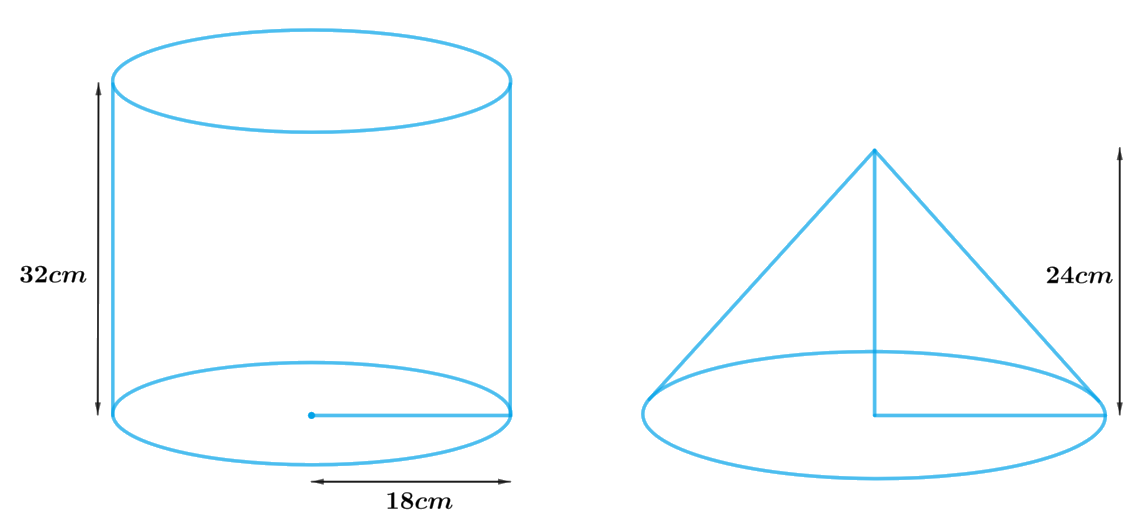# Ex.13.3 Q7 Surface Areas and Volumes Solution - NCERT Maths Class 10

## Question

A cylindrical bucket, $$32\,\rm{cm}$$ high and with radius of base $$18\,\rm{cm}$$, is filled with sand. This bucket is emptied on the ground and a conical heap of sand is formed. If the height of the conical heap is $$24\,\rm{cm}$$. Find the radius and slant height of the heap.

Video Solution
Surface Areas And Volumes
Ex 13.3 | Question 7

## Text Solution

What is known?

Height of the cylindrical bucket is $$32\rm{cm}$$ and radius is $$18\rm{cm.}$$

Height of the conical heap is $$24\rm{cm}$$

What is unknown?

The radius and slant height of the heap.

Reasoning:

Draw a figure to visualize the shapes betterSince, a cylindrical bucket filled with sand, emptied on the ground and a conical heap of sand is formed. Then the volume of sand in the cylindrical bucket will be same as the volume the conical heap of sand.

Therefore, volume the conical heap of sand $$=$$ volume of sand in the cylindrical bucket

We will find the volume of the sand by using formulae;

Volume of the cylinder $$= \pi {r^2}h$$

where $$r$$ and $$h$$ are the radius and height of the cylinder respectively

Volume of the cone \begin{align}= \frac{1}{3}\pi {r^2}h\end{align}

$l = \sqrt {{r^2} + {h^2}}$

where $$r, h$$ and $$l$$ are radius, height and slant height of cone respectively.

steps:

Height of the cylindrical bucket,$${h_1} = 32 \rm cm$$

Radius of the cylindrical bucket,$${r_1} = 18 \rm cm$$

Height the of conical heap, $$h = 24{\rm{ cm}}$$

Let the radius of the conical heap be $$r$$ and slant height be $$l.$$

volume the conical heap of sand $$=$$ volume of sand in the cylindrical bucket

\begin{align}\frac{1}{3}\pi {r^2}h &= \pi {r_1}^2{h_1}\\\frac{1}{3}\pi {r^2}h &= \pi {r_1}^2{h_1}\\ {r^2} &= \frac{{3\pi {r_1}^2{h_1}}}{h}\\{r^2} &= \frac{{ \begin{Bmatrix} 3 \times {{\left( {18 \rm cm} \right)}^2} \times \\ 18 \rm cm \times 32 \rm cm \end{Bmatrix} }}{{24 \rm cm}}\\r &= \sqrt {{{\left( {18 \rm cm} \right)}^2} \times 4} \\r &= 18 \rm cm \times 2\\\,\,r\, &= 36\,\rm cm\end{align}

Slant height, $$l = \sqrt {{r^2} + {h^2}}$$

\begin{align}&= \sqrt {{{\left( {36 \rm cm} \right)}^2} + {{\left( {24 \rm cm} \right)}^2}} \\&= \sqrt {1296 \rm c{m^2} + 576 \rm c{m^2}} \\&= \sqrt {1872 \rm c{m^2}} \\&= 12\sqrt {13} \rm cm\end{align}

Therefore, the radius and slant height of the conical heap are $$36\,\rm{cm}$$ and $${\text{12}}\sqrt {{\text{13}}\,} \,{\text{cm}}$$  respectively.

Learn from the best math teachers and top your exams

• Live one on one classroom and doubt clearing
• Practice worksheets in and after class for conceptual clarity
• Personalized curriculum to keep up with school